Math Concepts

# Rational Function

3.6k views

 1 Introduction 2 Rational Function 3 Domain and Range of Rational function 4 Inverse of a Rational Function 5 Properties of rational functions 6 Summary 7 FAQs

16 October  2020

## Introduction

What is a rational function?

A rational function is an algebraic fraction with numerator and denominator as polynomials and the denominator is not equal to zero.
The coefficients of the polynomials need not be rational numbers.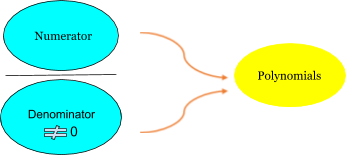Can you introduce a rational number with examples?

##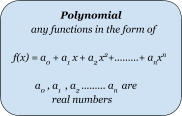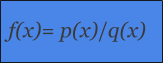### Rational function definition

As seen from the visual representation, R(x) will be a rational function and is a rational expression if p(x) and q(x) are polynomials.

Rational Function Examples: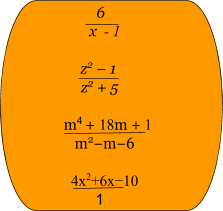## Domain and Range of Rational function

In a function f (x),

Domain of  f ---- The set of all real numbers that variable x  takes such that the expression defining the function is real. The domain can also be given explicitly.

The set of all values that f takes ---the  range  of the rational functions

Before moving on, let us recall that

• ℕ is the set of natural numbers.
• ℤ is the set of integers.
• ℚ is the set of rational numbers.
• ℝ is the set of real numbers.
• ℂ is the set of complex numbers.

Rational functions are functions of the form  f(x) = p(x)/q(x)

And we know p(x) and q(x) are polynomials with q(x) not being equal to 0.

Thus, this particular condition helps find the domain of rational function.

To find the domain of rational function find out the values of x for which denominator is 0. Then exclude these values.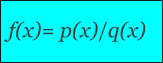### How to find the domain of a rational function?

1. where  p(x) and  q(x)  are polynomials and q(x)≠0
2. The domain of a rational function consists of all the real numbers x except those for which the denominator is 0.
3. To find these x values to be excluded from the domain of a rational function, equate the denominator to zero and solve for x

For example, The domain of the rational function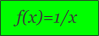is the set of all real numbers except x = 0.

 Example 2

Now, consider the rational function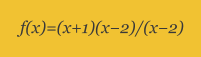On simplification, when x≠2 it becomes a linear function  f(x)=x+1

But at x=2, the original rational expression is not defined and hence it leaves the graph with a hole when x=2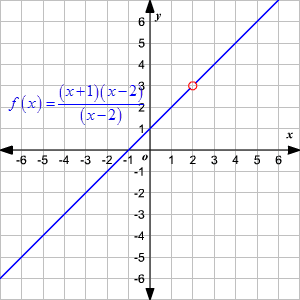How to find the range of a rational function:

1. Finding domain of the inverse rational function.
2. Sketch the rational function graph and identify the range.

Let us again consider the rational parent function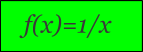1. We know that the function is not defined at x=0
2. As  x→0 from either side of zero,  f(x)→∞  Similarly, as   x→±∞,  f(x)→0
3. The graph approaches the x-axis as  when x tends to move towards (+ ∞)
4. or (- ∞,) but never touches the x-axis.
5. It  implies that the function can take all the real values except 0.
6. Hence the range of the function is the set of real numbers except 0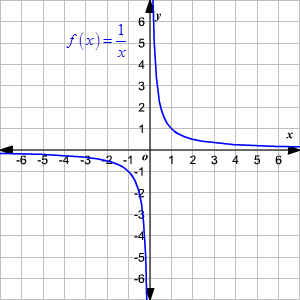Example 1

How to find the domain of a rational function? How to find the range of a rational function: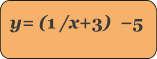In order to find the excluded value in the domain of the rational function,

Consider the denominator  equals to 0 and then  solve for x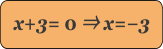This way we can find the domain of the rational function as a set of real numbers except −3

The range of the rational function is the same as the domain of the inverse rational function. So, to find the range define the inverse of the rational function.

Interchange the x and y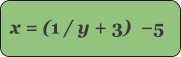Solving for y you get,

x + 5 = 1/y + 3

⇒ y + 3  = 1 / x + 5
⇒ y = (1 / x + 5) − 3

So, the inverse rational function is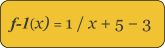We can find excluded value in the domain of the inverse rational expression by making the denominator  equals to zero and  then solving for x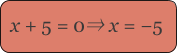Hence the domain of the inverse rational function is the set of real numbers except −5. It means that the range of the given function is the set of real numbers except −5

Therefore, the domain of the given rational function is

{x ∈ R  |  x ≠ −3} and the range is  {y ∈ R  |  y ≠ −5}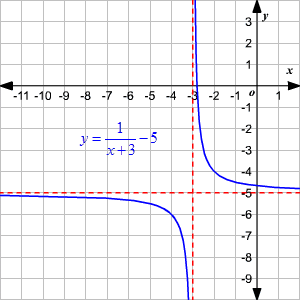How to find the domain of a rational function, How to find the range of a rational function with one unknown in the denominator.

 Example 2

Find the domain and range of the rational function f(x) = -1/x-5.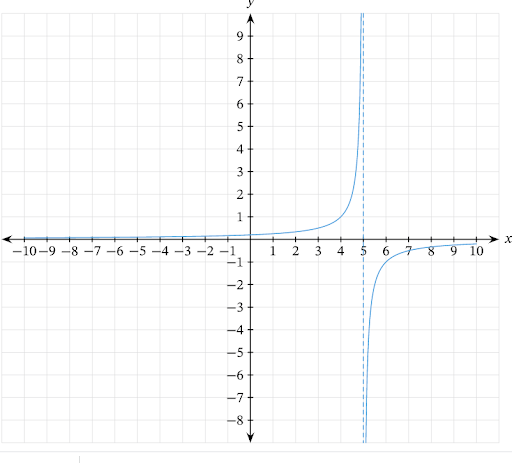### Domain of a Rational function:

• From the above given graph it implies that the domain = ℝ−{5} and the Range = ℝ−{0}. We need to check this algebraically.
• A rational function is not defined for any set of values that results in a zero denominator. Equate the denominator to zero    as , 𝑥−5=0, which gives a solution of  𝑥=5.
• Hence it confirms as the domain is ℝ−{5}.

### How to find the range of a rational function?

• To confirm the range, identify the set of values that cannot be achieved given the domain. As the  magnitude of 𝑥 gets larger, the output tends to zero but will never  reach 0. Therefore, the range = ℝ−{0}.
 Example 3

Find the domain and range of the rational expression 1/x-2.

### Solution:

• Follow the same procedure as in previous example
• Domain of a Rational Function: Solve for
•                  𝑥−2=0,
• Therefore, the domain =  ℝ−{2}.
•
• For range of a rational function, the  magnitude of 𝑥 gets larger, the output tends to zero but will never  reach 0. Therefore, the range = ℝ−{0}.
 Example 4

Find the domain and range of the rational function  f(x) = 2x+3/4x+5 and also find values that f(X) cannot take.

### Domain of a rational Function

𝑓(𝑥) is undefined when the denominator value = 0.

Hence, at   4𝑥+5=0  , the graph of the function would have an asymptote.

To find the equation of asymptote,follow the below steps:
4𝑥+5-5=-5
𝑥 = −5 / 4
Therefore, the asymptote has the equation 𝑥 = −5 / 4.

And the domain of the function is all real numbers except −5 / 4 ,i.e., ℝ−{ − 5 /4}.

### How to find the range of a rational function?

Values 𝑓(𝑥) cannot take, it helps to find what happens as 𝑥 gets large. To make this process easier divide the numerator and denominator of the function by 𝑥 to get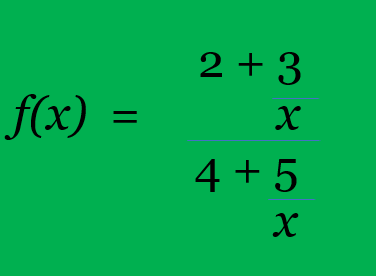### From this it’s clear that as 𝑥 gets progressively large, 3𝑥 and 5𝑥 tends closer to zero, and also the function tends to ½ but never actually reach it.  Range is R - {½}. Asymptotes of a rational function

Let's check out one of our old friends...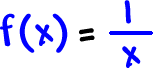See how the graph is getting closer to the axes?...  But, it will never touch them?
The x and y axes are asymptotes!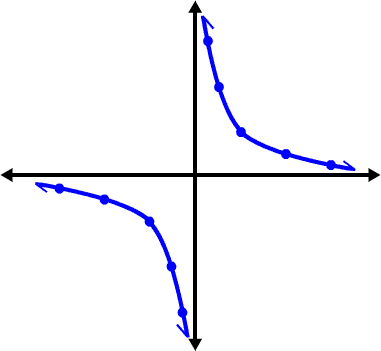Asymptotes are lines (usually invisible) in space that a graph gets closer and closer to but never touches...

So for vertical asymptotes...Set the denominator = 0 and solve.

## Inverse of a Rational Function

Finding the inverse of a rational function is relatively easy,because  the procedure to find the inverse is rather repetitious. That the method used here is just one way out of the many.

Feel free to vary the steps to fit your needs as long as you’re getting the same final answer.

Key Steps in Finding the Inverse of a Rational Function

1. Replace f(x)  by y.
2. Switch the roles of x and y, in other words, interchange x and y in the equation.
3. Solve for y in terms of x.
4. Replace y by f−1(x)  to get the inverse function.

### Examples of How to Find the Inverse of a Rational Function

 Example 1

Find the inverse function. State its domain and range.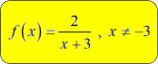Even without graphing this function,  x cannot equal -3  .Because the denominator becomes zero, and the entire rational expression becomes undefined. The domain is all x−values not including −3.

Next, let's graph this function to verify if it passes the Horizontal Line Test, this guarantees to have an inverse function.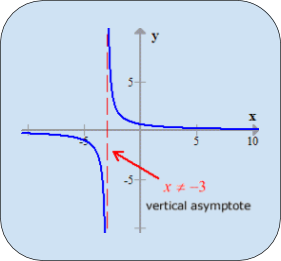It seems that no horizontal line will intersect the graph more than once. That’s a good sign. The vertical dashed line is called a vertical asymptote. The graph will never cross that. Remember, it is coming from the zeros of the denominator, or the restricted values of x.

Using the above steps, let’s find the inverse.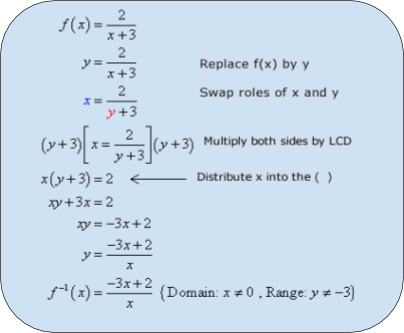The domain of the inverse function comes from the fact that the denominator cannot equal zero. The range is obtained from the domain of the original function.

 Example 2

Find the inverse function. State its domain and range.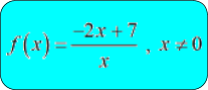This is the graph of the original function.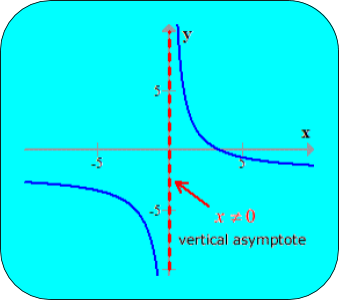The following are the steps to find the inverse.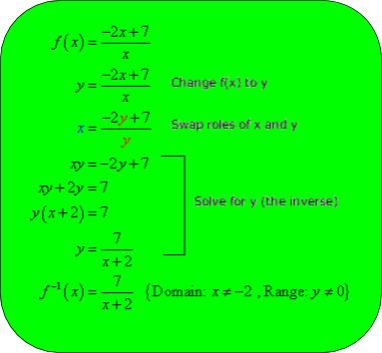Example 3

Find the inverse function.  State its domain and range.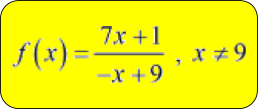.

In this problem since the rational expression has linear terms in both numerator and denominator, be convinced that just like in the previous example, it will have an inverse function even without further testing. Add it to your math toolbox and utilize it as your advantage whenever you encounter similar problems. Just to verify, the graph is shown below.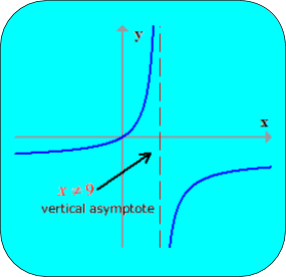The rest of the steps to find the inverse function are the same. At the end of the solution, to get the final answer do factoring and then canceling the hidden −1, found in both numerator and denominator.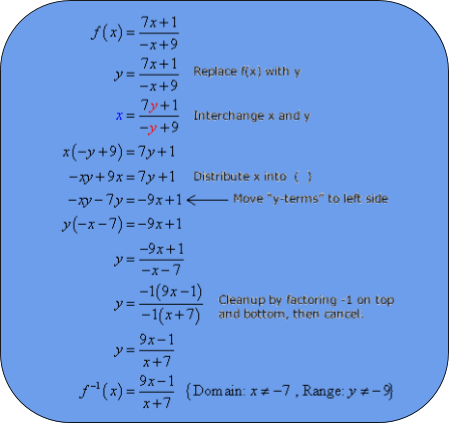## Properties of rational functions.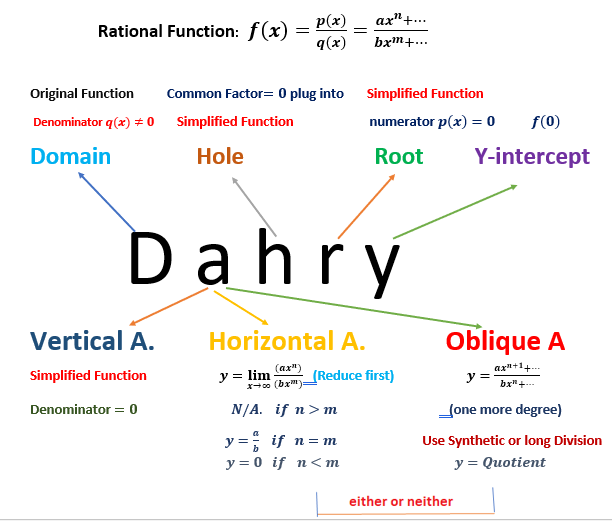The following are general Properties of rational functions:

• If the numerator and denominator are of the same degree (n=m), then y= an/bm  is a horizontal asymptote of the function.
• If the degree of the denominator is greater than the degree of the numerator, then y=0 is a Horizontal asymptote.
• If the degree of the denominator is less than the degree of the numerator, then there are no horizontal asymptotes.
• When x is equal to a root of the denominator polynomial, the denominator is zero and there is a Vertical asymptote. The exception is the case when the root of the denominator is also a root of the numerator. However, for  this case we can cancel a factor from both the numerator and denominator (and we effectively have a lower-degree rational function).

## Summary:

A rational function is an algebraic fraction with numerator and denominator as polynomials and the denominator is not equal to zero.
The coefficients of the polynomials need not be rational numbers.

How to find the domain of a rational function?

The domain of a rational function consists of all the real numbers x except those for which the denominator is 0.
To find these x values to be excluded from the domain of a rational function, equate the denominator to zero and solve for x

How to find the range of a rational function:

• Finding domain of the inverse of rational function.
• Sketch the rational function graph and identify the range.

We also discussed  properties of rational functions.

Written by Asha M.

## What is a rational function?

A rational function is an algebraic fraction with numerator and denominator as polynomials and the denominator is not equal to zero.

The coefficients of the polynomials need not be rational numbers.

## How to find the domain of a rational function?

where  p(x) and  q(x)  are polynomials and q(x)≠0

The domain of a rational function consists of all the real numbers x except those for which the denominator is 0.

To find these x values to be excluded from the domain of a rational function, equate the denominator to zero and solve for x

Related Articles
GIVE YOUR CHILD THE CUEMATH EDGE
Access Personalised Math learning through interactive worksheets, gamified concepts and grade-wise courses